In astrodynamics and celestial mechanics a radial trajectory is a Kepler orbit with zero angular momentum. Two objects in a radial trajectory move directly towards or away from each other in a straight line.

## Classification

There are three types of radial trajectories (orbits).

• Radial elliptic trajectory: an orbit corresponding to the part of a degenerate ellipse from the moment the bodies touch each other and move away from each other until they touch each other again. The relative speed of the two objects is less than the escape velocity. This is an elliptic orbit with semi-minor axis = 0 and eccentricity = 1. Although the eccentricity is 1 this is not a parabolic orbit. If the coefficient of restitution of the two bodies is 1 (perfectly elastic) this orbit is periodic. If the coefficient of restitution is less than 1 (inelastic) this orbit is non-periodic.
• Radial parabolic trajectory, a non-periodic orbit where the relative speed of the two objects is always equal to the escape velocity. There are two cases: the bodies move away from each other or towards each other.
• Radial hyperbolic trajectory: a non-periodic orbit where the relative speed of the two objects always exceeds the escape velocity. There are two cases: the bodies move away from each other or towards each other. This is a hyperbolic orbit with semi-minor axis = 0 and eccentricity = 1. Although the eccentricity is 1 this is not a parabolic orbit.

Unlike standard orbits which are classified by their orbital eccentricity, radial orbits are classified by their specific orbital energy, the constant sum of the total kinetic and potential energy, divided by the reduced mass: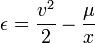$\epsilon = \frac{v^2}{2} - \frac{ \mu }{ x }$

where x is the distance between the centers of the masses, v is the relative velocity, and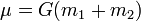$\mu={G}(m_1 + m_2)$ is the standard gravitational parameter.

Another constant is given by: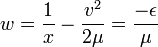$w = \frac{1}{x} - \frac{ v^2 }{ 2 \mu } = \frac{- \epsilon}{\mu}$
• For elliptic trajectories, w is positive. It is the inverse of the apoapsis distance (maximum distance).
• For parabolic trajectories, w is zero.
• For hyperbolic trajectories, w is negative, It is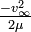$\textstyle \frac{ -v_{\infty }^2}{2 \mu}$ where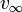$\textstyle v_{\infty }$ is the velocity at infinite distance.

## Time as a function of distance

Given the separation and velocity at any time, and the total mass, it is possible to determine the position at any other time.

The first step is to determine the constant w. Use the sign of w to determine the orbit type.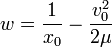$w = \frac{1}{ x_0 } - \frac{ v_0^2 }{ 2 \mu }$

where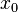$\textstyle x_0$ and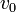$\textstyle v_0$ are the separation and relative velocity at any time.

### Parabolic trajectory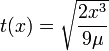$t(x) = \sqrt{ \frac{2 x^3 }{ 9 \mu } }$

where t is the time from or until the time at which the two masses, if they were point masses, would coincide, and x is the separation.

This equation applies only to radial parabolic trajectories, for general parabolic trajectories see Barker's equation.

### Elliptic trajectory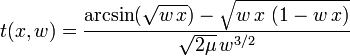$t( x,w ) = \frac{ \arcsin( \sqrt{ w \, x } ) - \sqrt{ w \, x \ ( 1 - w \, x ) } }{ \sqrt{ 2 \mu } \, w^{3/2} }$

where t is the time from or until the time at which the two masses, if they were point masses, would coincide, and x is the separation.

This is the radial Kepler equation.

### Hyperbolic trajectory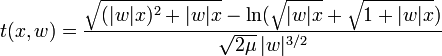$t( x,w ) = \frac{ \sqrt{ (|w|x)^2+|w|x} - \ln (\sqrt{|w|x}+\sqrt{1+|w|x}) }{ \sqrt{ 2 \mu } \, |w|^{3/2} }$

where t is the time from or until the time at which the two masses, if they were point masses, would coincide, and x is the separation.

### Universal form (any trajectory)

The radial Kepler equation can be made "universal" (applicable to all trajectories):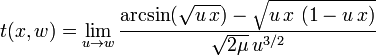$t( x, w ) = \lim_{ u \to w } \frac{ \arcsin( \sqrt{ u \, x } ) - \sqrt{ u \, x \ ( 1 - u \, x ) } }{ \sqrt{ 2 \mu } \, u^{3/2} }$

or by expanding in a power series: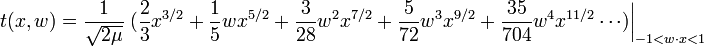$t( x, w ) = \frac{ 1 }{ \sqrt{ 2 \mu } } \left. (\frac{2}{3} x^{3/2} + \frac{1}{5} w x^{5/2} + \frac{3}{28} w^2 x^{7/2} + \frac{5}{72} w^3 x^{9/2} + \frac{35 }{704 } w^4 x^{11/2} \cdots ) \right|_{ -1

## The radial Kepler problem (distance as function of time)

The problem of finding the separation of two bodies at a given time, given their separation and velocity at another time, is known as the Kepler problem. This section solves the Kepler problem for radial orbits.

The first step is to determine the constant w. Use the sign of w to determine the orbit type.$w = \frac{1}{ x_0 } - \frac{ v_0^2 }{ 2 \mu }$

Where$\textstyle x_0$ and$\textstyle v_0$ are the separation and velocity at any time.

### Parabolic trajectory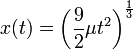$x(t) = \left( \frac{9}{2} \mu t^2 \right)^{ \frac{1}{3} }$

### Universal form (any trajectory)

Two intermediate quantities are used: w, and the separation at time t the bodies would have if they were on a parabolic trajectory, p.$w = \frac{1}{x_0} - \frac{ v_0^2 }{ 2 \mu } \quad \text{and} \quad p = \left( \frac{9}{2} \mu t^2 \right)^{ \frac{1}{3} }$

Where t is the time,$x_0$ is the initial position,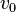$v_0$ is the initial velocity, and$\mu ={G}(m_1 + m_2)$.

The inverse radial Kepler equation is the solution to the radial Kepler problem: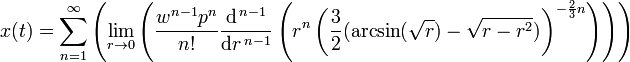$x( t ) = \sum_{n=1}^{ \infty } \left( \lim_{ r \to 0 } \left( {\frac{ w^{n-1} p^{ n }}{ n! }} \frac{\mathrm{d}^{\,n-1}}{\mathrm{ d } r ^{\,n-1}} \left( r^n \left( \frac{ 3 }{ 2 } ( \arcsin( \sqrt{ r } ) - \sqrt{ r - r^2 } ) \right)^{ - \frac{2}{3} n } \right) \right) \right)$

Evaluating this yields: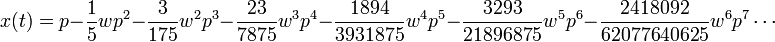$x(t) = p - \frac{1}{5} w p^2 - \frac{3}{175} w^2 p^3 - \frac{23}{7875} w^3 p^4 - \frac{1894}{3931875} w^4 p^5 - \frac{3293}{21896875} w^5 p^6 - \frac{2418092}{62077640625}w^6 p^7 \cdots$

Power series can be easily differentiated term by term. Repeated differentiation gives the formulas for the velocity, acceleration, jerk, snap, etc.

## Orbit inside a radial shaft

The orbit inside a radial shaft in a uniform spherical body would be a simple harmonic motion, because gravity inside such a body is proportional to the distance to the center. If the small body enters and/or exits the large body at its surface the orbit changes from or to one of those discussed above. For example, if the shaft extends from surface to surface a closed orbit is possible consisting of parts of two cycles of simple harmonic motion and parts of two different (but symmetric) radial elliptic orbits.